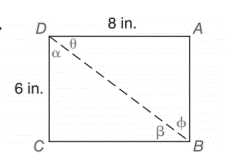Chapter 11.2, Problem 28EElementary Geometry For College St...

7th Edition
Alexander + 2 others
ISBN: 9781337614085

Solutions

Chapter
SectionElementary Geometry For College St...

7th Edition
Alexander + 2 others
ISBN: 9781337614085
Textbook Problem

In Exercise 23 to 28, use either the sine ratio or the cosine ratio as needed to find the measure of each indicated angle to the nearest degree.To determine

To find:

The measure of each indicated angle of the following triangle,Explanation

Consider the following figure,

General formula for cosine ratio and sin ratio is given below,

In a rectangle opposite sides are equal. That is, AD=BC=8in.andCD=AB=6in.

The Pythagorean theorem is given below,

(BD)2=(CD)2+(BC)2

Substitute the values of AD=BC=8in.andCD=AB=6in. to get the following,

(BD)2=(6)2+(8)2(BD)2=36+64(BD)2=100BD=10in.

Hence 10 in. is the length of leg hypotenuse.

From the given figure the value 10 in. is the hypotenuse value since it is opposite to right angle.

Then 6 in. is the length of the leg adjacent to α and 6 in. is the length of leg opposite to β. Then 8 in. is the length of the leg adjacent to θ and 8 in. is the length of leg opposite to ϕ.

Therefore,

cosα=610cosα=35α=cos1(35)

Using a graphing calculator in degree mode, follow the following key sequence,

cos1(3/5)enter53

So the value of α is given below,

α=53

Therefore,

Still sussing out bartleby?

Check out a sample textbook solution.

See a sample solution

The Solution to Your Study Problems

Bartleby provides explanations to thousands of textbook problems written by our experts, many with advanced degrees!

Get Started

Find more solutions based on key concepts